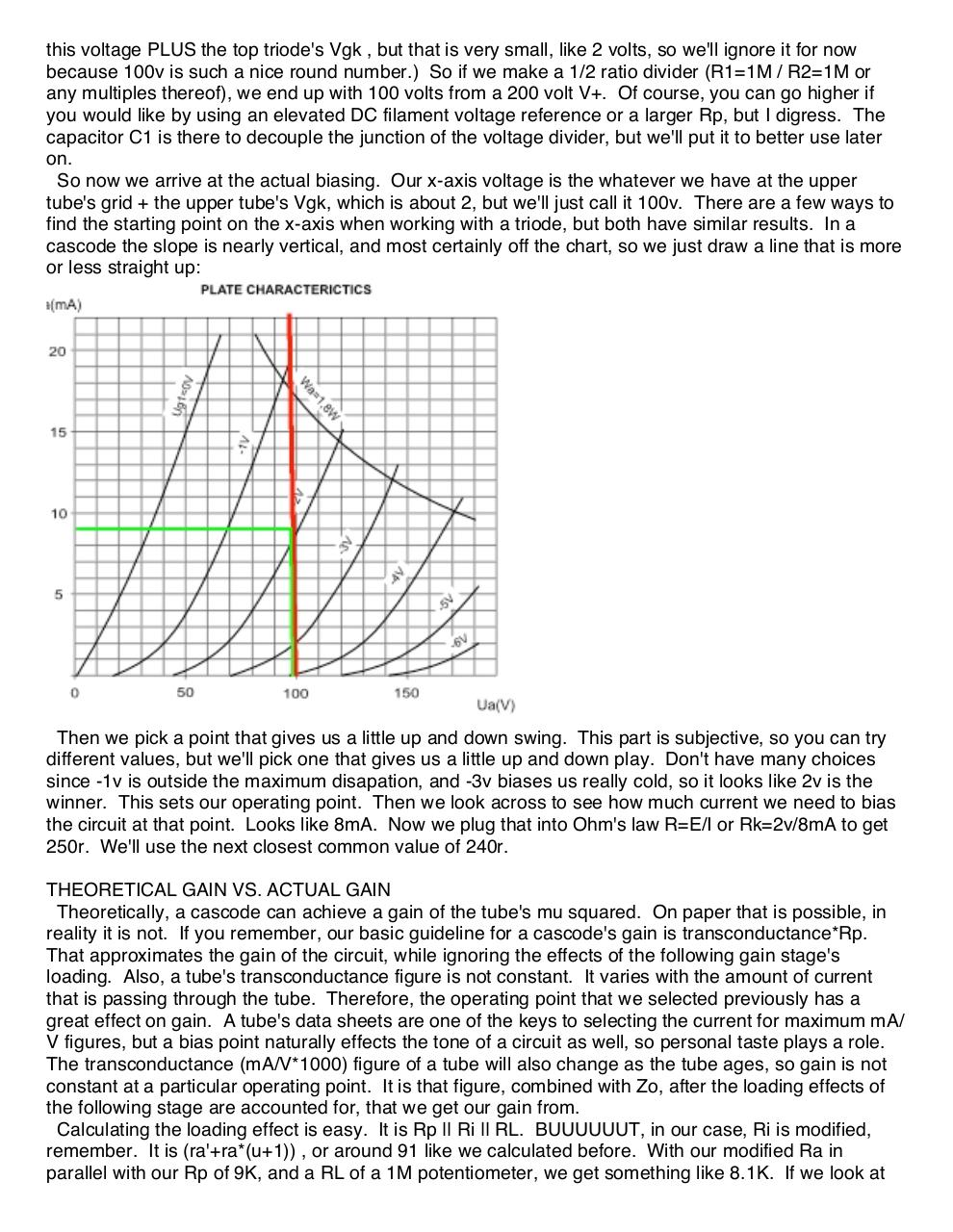# alex kenis cascodes.pdfPage 1 2 3 4 5 6

#### Text preview

this voltage PLUS the top triode's Vgk , but that is very small, like 2 volts, so we'll ignore it for now
because 100v is such a nice round number.) So if we make a 1/2 ratio divider (R1=1M / R2=1M or
any multiples thereof), we end up with 100 volts from a 200 volt V+. Of course, you can go higher if
you would like by using an elevated DC filament voltage reference or a larger Rp, but I digress. The
capacitor C1 is there to decouple the junction of the voltage divider, but we'll put it to better use later
on.
So now we arrive at the actual biasing. Our x-axis voltage is the whatever we have at the upper
tube's grid + the upper tube's Vgk, which is about 2, but we'll just call it 100v. There are a few ways to
find the starting point on the x-axis when working with a triode, but both have similar results. In a
cascode the slope is nearly vertical, and most certainly off the chart, so we just draw a line that is more
or less straight up:

Then we pick a point that gives us a little up and down swing. This part is subjective, so you can try
different values, but we'll pick one that gives us a little up and down play. Don't have many choices
since -1v is outside the maximum disapation, and -3v biases us really cold, so it looks like 2v is the
winner. This sets our operating point. Then we look across to see how much current we need to bias
the circuit at that point. Looks like 8mA. Now we plug that into Ohm's law R=E/I or Rk=2v/8mA to get
250r. We'll use the next closest common value of 240r.
THEORETICAL GAIN VS. ACTUAL GAIN
Theoretically, a cascode can achieve a gain of the tube's mu squared. On paper that is possible, in
reality it is not. If you remember, our basic guideline for a cascode's gain is transconductance*Rp.
That approximates the gain of the circuit, while ignoring the effects of the following gain stage's
loading. Also, a tube's transconductance figure is not constant. It varies with the amount of current
that is passing through the tube. Therefore, the operating point that we selected previously has a
great effect on gain. A tube's data sheets are one of the keys to selecting the current for maximum mA/
V figures, but a bias point naturally effects the tone of a circuit as well, so personal taste plays a role.
The transconductance (mA/V*1000) figure of a tube will also change as the tube ages, so gain is not
constant at a particular operating point. It is that figure, combined with Zo, after the loading effects of
the following stage are accounted for, that we get our gain from.
Calculating the loading effect is easy. It is Rp || Ri || RL. BUUUUUUT, in our case, Ri is modified,
remember. It is (ra'+ra*(u+1)) , or around 91 like we calculated before. With our modified Ra in
parallel with our Rp of 9K, and a RL of a 1M potentiometer, we get something like 8.1K. If we look at